# CHEMICAL REACTIONS Describing Chemical Change Objectives Write equations

• Slides: 10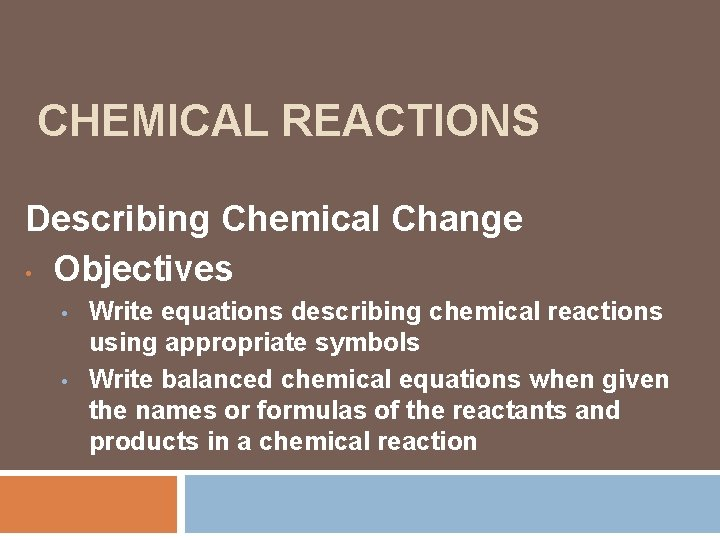CHEMICAL REACTIONS Describing Chemical Change • Objectives • • Write equations describing chemical reactions using appropriate symbols Write balanced chemical equations when given the names or formulas of the reactants and products in a chemical reaction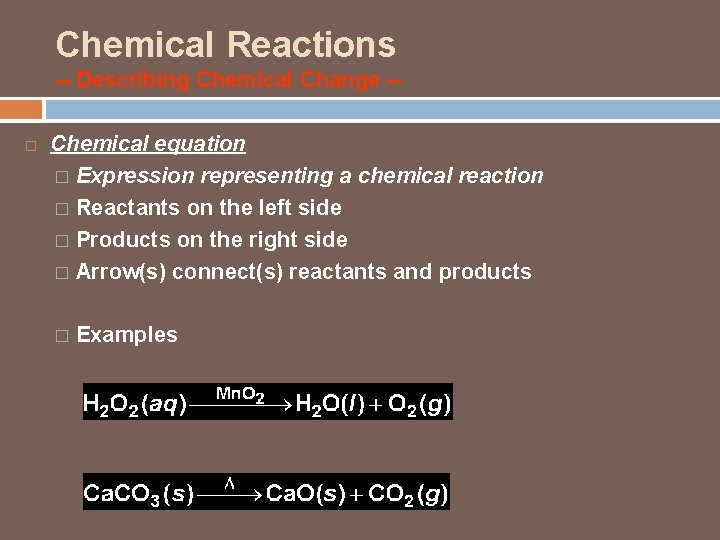Chemical Reactions -- Describing Chemical Change - Chemical equation � Expression representing a chemical reaction � Reactants on the left side � Products on the right side � Arrow(s) connect(s) reactants and products � Examples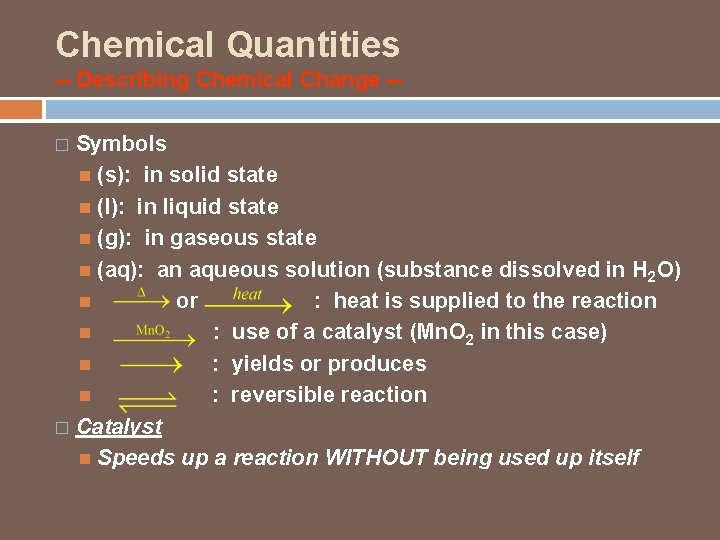Chemical Quantities -- Describing Chemical Change -Symbols (s): in solid state (l): in liquid state (g): in gaseous state (aq): an aqueous solution (substance dissolved in H 2 O) or : heat is supplied to the reaction : use of a catalyst (Mn. O 2 in this case) : yields or produces : reversible reaction � Catalyst Speeds up a reaction WITHOUT being used up itself �Chemical Reactions -- Describing Chemical Change -- � Skeleton equation Chemical equation that does not indicate the relative amounts of reactants and products Does not necessarily follow the Law of Conservation of Mass What does this law state? Example: Frame + Wheel + Handle bar + Pedal Bicycle Write an example of your own. Raise your hand have me come check it when you are finished.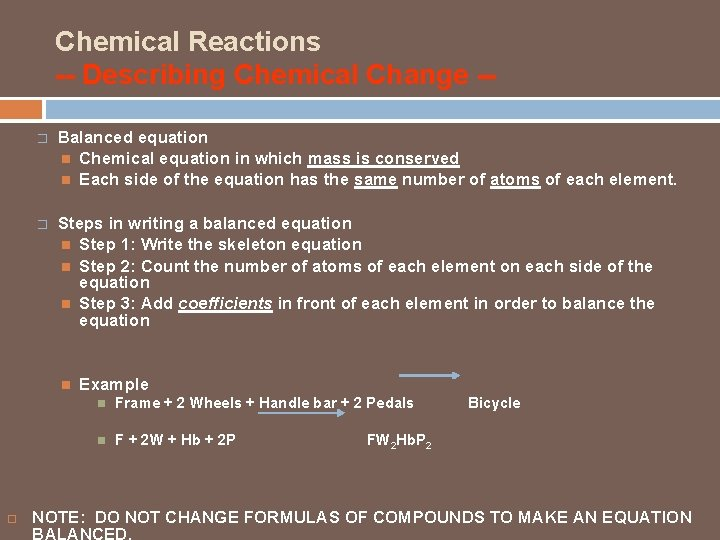Chemical Reactions -- Describing Chemical Change -� Balanced equation Chemical equation in which mass is conserved Each side of the equation has the same number of atoms of each element. � Steps in writing a balanced equation Step 1: Write the skeleton equation Step 2: Count the number of atoms of each element on each side of the equation Step 3: Add coefficients in front of each element in order to balance the equation Example Frame + 2 Wheels + Handle bar + 2 Pedals F + 2 W + Hb + 2 P Bicycle FW 2 Hb. P 2 NOTE: DO NOT CHANGE FORMULAS OF COMPOUNDS TO MAKE AN EQUATION BALANCED.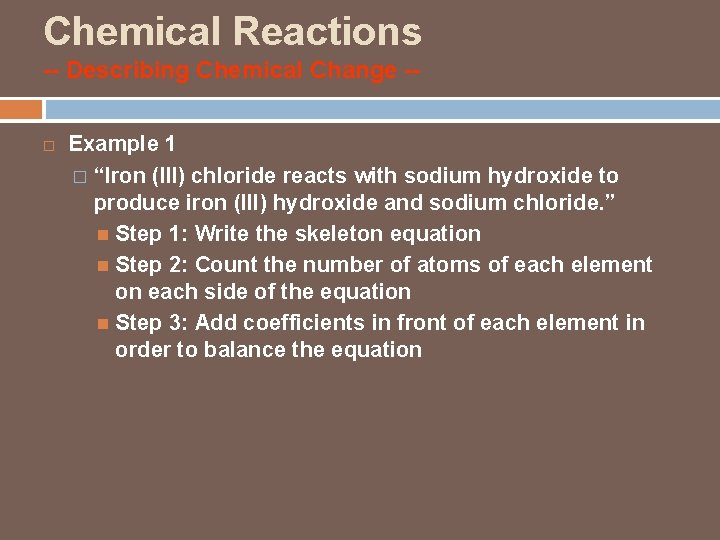Chemical Reactions -- Describing Chemical Change - Example 1 � “Iron (III) chloride reacts with sodium hydroxide to produce iron (III) hydroxide and sodium chloride. ” Step 1: Write the skeleton equation Step 2: Count the number of atoms of each element on each side of the equation Step 3: Add coefficients in front of each element in order to balance the equation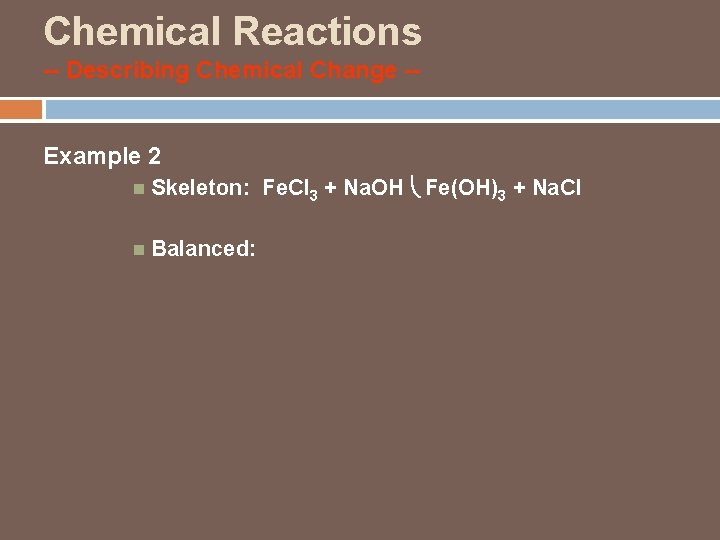Chemical Reactions -- Describing Chemical Change -Example 2 Skeleton: Fe. Cl 3 + Na. OH Fe(OH)3 + Na. Cl Balanced:Chemical Reactions -- Describing Chemical Change -Example 3 � “Copper (II) sulfide reacts with oxygen gas to produce copper and sulfur dioxide. ” Write the skeleton equation for the above reaction. Balance the above reaction. (Don’t forget to count the atoms on each side of the equation)Chemical Reactions -- Describing Chemical Change -- Example 4 Skeleton: Cu. S + O 2 Cu + SO 2 Balanced:Chem balancer Directions Directions: 1. Start Internet Explorer and in the search bar type “chem balancer” 2. Click on the first link “Classic Chembalancer” 2. Click ‘Directions’. Read and understand the directions. 3. Click ‘OK’. 4. Click on ‘Start Game’ 5. Try entering some numbers in the text boxes in front of each molecule. What happens?